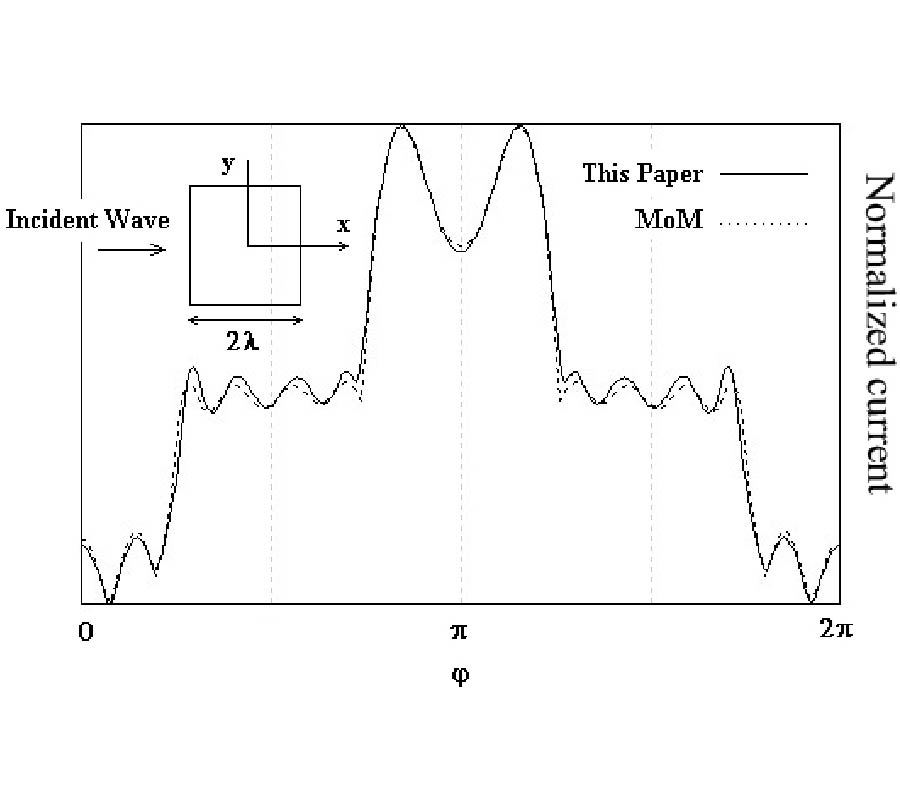# Vol. 7

Front:[PDF file] Back:[PDF file]
Latest Volume
All Volumes
All Issues
2009-06-18

#### A New Integral Equation Formulation for Scattering of Electromagnetic Waves by 2D Conducting Structures, Using Cylindrical Harmonics

By Nima Chamanara
Progress In Electromagnetics Research M, Vol. 7, 165-177, 2009
doi:10.2528/PIERM09042106

## Abstract

Using cylindrical harmonics and Fourier series, a new integral equation formulation is derived for perfectly conducting 2D scattering problems. This new integral equation is based on the fact that, all of the electric and magnetic field components are zero inside a perfect electric conductor. The incident and scattered fields are expressed in the cylindrical coordinate system with respect to a common origin inside the scatterer, using the addition theorem for Bessel and Hankel functions. The resulting electric or magnetic field is set equal to zero for all the points inside the largest cylinder that is contained in and tangent to the surface of the scatterer. As a result the field point variables are eliminated from the integral equation and only the source points are present in this formulation. Therefore the size of the problem is reduced considerably. A dramatic improvement in the computation speed is seen compared to the classical method of moments. TE and TM scattering problems are considered and the integral equation formulation is derived and solved for both cases.

## Citation

Nima Chamanara, "A New Integral Equation Formulation for Scattering of Electromagnetic Waves by 2D Conducting Structures, Using Cylindrical Harmonics," Progress In Electromagnetics Research M, Vol. 7, 165-177, 2009.
doi:10.2528/PIERM09042106
http://www.jpier.org/PIERM/pier.php?paper=09042106

## References

1. Saad, Y. and M. Schultz, "Gmres: A generalized minimal residual algorithm for solving nonsymmetric linear systems," SIAM J. Sci. Statist. Comput., Vol. 7, 856-869, 1986.
doi:10.1137/0907058

2. Concus, P., G. Golub, and D. O'Leary, "Generalized conjugate gradient method for the numerical solution of elliptic partial di®erential equations," Sparse Matrix Computations, 309-332, Academic, New York, 1976.

3. Michielssen, E. and A. Boag, "Multilevel evaluation of electromagnetic ¯elds for the rapid solution of scattering problems," Microwave Opt. Technol. Lett., Vol. 7, No. 17, 790-795, 1994.
doi:10.1002/mop.4650071707

4. Coifman, R., V. Rokhlin, and S. Wandzura, "The fast multipole method for the wave equation: A pedestrian prescription," IEEE Antennas Propagat. Mag., Vol. 35, 7-12, 1993.
doi:10.1109/74.250128

5. Song, J. M. and W. C. Chew, "Fast multipole method solution using parametric geometry," Microwave Opt. Technol. Lett., Vol. 7, No. 16, 760-765, 1994.
doi:10.1002/mop.4650071612